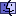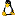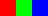Understanding ImageData, MaskData and AlphaDataUPDATE 7/29/02: The MetaCard 2.4.3 engine (used by MetaCard 2.4.3 and Revolution 1.5A7 or later) fixed a bug that was identified in the previous version of this Tip relating to the order of the color bytes in `imageData`.

 The Tip on this page is based on the MetaCard 2.4.3 engine. If you have a version of MetaCard/Revolution that uses an earlier engine than 2.4.3, you can view the original Tip for assistance.

UPDATE 9/12/02: Fixed examples to make sure byte order is correct.

The Basics

Each image object can store an image, for example, when you import one from disk or create one using MC/Rev’s paint tools.
Pixels: The actual image in each image object can be described as having X * Y pixels, where X is the width of the image and Y is the height. That is, a 20 x 40 image has 800 (20 x 40 = 800) pixels.
Bits: The image is composed of a sequence of 32-bit binary values (key in on the word binary), so each pixel in an contains 32 bits; eight for red, eight for green, eight for blue and eight for something else (an alpha value, I think. I’m going to call it “Other” for the purposes of this tip. — Ed.). An example of the binary value for 129 is 10000001.
Bytes: There are eight bits in a byte, so each pixel is composed of four bytes. Each byte can represent a value from 0 to 255 (00000000 = 0, 11111111 = 255).
Based on the above, our 20 x 40 image has these stats:

 Type Calculation Total Pixels `40 x 20 =` `800` Bytes `40 x 20 x 4 =` `3,200` Bits `40 x 20 x 4 x 8 =` `25,600`

Byte Order: Each pixel is represented by four bytes in the order of Other, Red, Green, Blue. The Other byte is always 0.

Example So Far

Using the basic information above, a three pixel (1 row by 3 pixels) image where the first pixel is pure Red, the second pure Green and the third pure Blue () would have the following:

 Red Pixel Green Pixel Blue Pixel Pixel 1 2 3 Byte `1` `2` `3` `4` `5` `6` `7` `8` `9` `10` `11` `12` Bit `Other` `Red` `Green` `Blue` `Other` `Red` `Green` `Blue` `Other` `Red` `Green` `Blue` Bit Value `00000000` `11111111` `00000000` `00000000` `00000000` `00000000` `11111111` `00000000` `00000000` `00000000` `00000000` `11111111` Byte Value `0` `255` `0` `0` `0` `0` `255` `0` `0` `0` `0` `255`

Retreiving Image Data

The `imageData` property: The 32-bit binary values are retrieved from an image by asking for the `imageData` of an image (and can be stored in a variable or custom property for later use). You can retrieve the bytes of imageData (remember there are 4 of them for each pixel) as an ASCII value by asking for the ```charToNum(char X of the imageData of imageDescriptor)```, where X is the byte number to retrieve.

 Why does this work? Well, if you notice that the byte values in the table above are able to contain a value from 0 to 255, and you consider that the ASCII value of a character is also from 0 to 255, it makes sense to be able to retrieve the numeric value of a byte by thinking of the bytes of an image as characters, and then using `charToNum` to get the value.

So in our 3-pixel image example above (which we’ll call "ThreePixel"), if you executed...

``` answer charToNum(char 6 of the imageData of image "ThreePixel") ```
... you would get 0 displayed in the Answer dialog, which would correspond to the byte value of the Red bit of the second pixel (the Green pixel) of our image.

Note also that if you...

``` answer length(the imageData of image "ThreePixel") ```
... you would get 12 displayed in the Answer dialog (because it shows the number of characters, or bytes in the `imageData`, and since there are 4 bytes of `imageData` for every pixel, you get 12 in the result).

The `maskData` property: This is a property of an image where it is an all-or-nothing mask of a given pixel of an image. Note that this is dealing with pixels, not bits. The `maskData` contains 8-bit (1 byte or character) binary values that have a value that is either zero or non-zero. If the value is 0, that pixel is transparent. If the value is non-zero, the pixel is opaque. You can retrieve the ASCII value of a pixel’s maskData in the same way you do with `imageData` above.

So in our 3-pixel image example, if you executed...

``` answer charToNum(char 1 of the maskData of image "ThreePixel") ```
... you would get `255` displayed in the Answer dialog, which would correspond to the default non-zero value for the mask of the Red pixel in our image. Since it is 255, the pixel is opaque. Note also that if you...
``` answer length(the maskData of image "ThreePixel") ```
... you would get 3 displayed in the Answer dialog because it shows the number of characters, or bytes in the `maskData`, and since there is 1 byte of `maskData` for every pixel, you get 3 in the result).

The `alphaData` property: This is a property of an image where you can impose a variable level of transparency for a given pixel of an image. Note that like `maskData`, this is dealing with pixels, not bits. The `alphaData` contains 8-bit (1 byte or character) binary values that have a value from 0 to 255. If the value is 0, the pixel is transparent. If the value is 255, the value is fully opaque. Any other value inbetween sets a level of transparency (i.e. 128 would make the pixel 50% transparent). You can retrieve the ASCII value of a pixel’s alphaData in the same way you do with `imageData` above.

So in our 3-pixel image example, if you executed...

``` answer charToNum(char 1 of the alphaData of image "ThreePixel") ```
... you would get `255` displayed in the Answer dialog, which would correspond to the fully opaque value for the alpha level of the Red pixel in our image.

 You probably noticed that it is similar to the `maskData` property, and can effectively do everything `maskData` can do and more. So why not just use `alphaData` all the time? The reason is that `alphaData` takes a lot longer to execute than `maskData`, so if you just want to turn pixels on and off, you’re better off using `maskData`.

Note also that if you...

``` answer length(the alphaData of image "ThreePixel") ```
... you would get 3 displayed in the Answer dialog because it shows the number of characters, or bytes in the `alphaData`, and since there is 1 byte of `alphaData` for every pixel, you get 3 in the result).

Setting Image Data
Keep in mind that you don’t set specific parts of `imageData`, `maskData` or `alphaData`, you set these properties as a whole. That is, let’s say you want to make the second row of pixels in an image to be transparent. You choose to set the `maskData` of these pixels to 0, and you’ll need to use the `binaryEncode` function in order to set the binary data (remember that all of this image information is stored in binary). Here’s a simple example that will do that:

```on mouseUp
put the imageData of img 1 into iData
put empty into mData
put the height of img 1 into tH
put the width of img 1 into tW
put binaryEncode("C",0) into transparentPixel
put binaryEncode("C",255) into opaquePixel
repeat with i = 1 to tH   -- iterate through each row
repeat with j = 1 to tW   -- iterate through each column
if i = 2 then
put transparentPixel after mData
else
put opaquePixel after mData
end if
end repeat
end repeat
set the maskData of img 1 to mData
set the imageData of img 1 to iData
end mouseUp```
You can see that the `maskData` was set as a whole.

 IMPORTANT! You need to set the `imageData` of the image after you have applied a mask or an alpha change. It doesn’t seem to work if you do it the other way around.

There are two basic uses of `binaryEncode` when setting `imageData`, `maskData`, or `alphaData`. In both of these instances, you are setting the equivalent of one pixel of the image.

The first is used to set `imageData`:

``` binaryEncode("CCCC",0,redByte,greenByte,blueByte) ```
The second is used to set `maskData` or `alphaData`:
``` binaryEncode("C",{maskOrAlphaByte}) ```
In the cases above, redByte, greenByte, blueByte, and maskOrAlphaByte each contain a value from 0 to 255.

To show you how this works, below is a script that creates a 20 x 20 pure blue image, that is set to 50% transparency:

```on mouseUp
create image
put it into tID
set the width of tID to 20
set the height of tID to 20
put empty into iData
put empty into aData
put binaryEncode("CCCC",0,0,0,255) into bluePixel
put binaryEncode("C",128) into halfTransPixel
repeat with i = 1 to 400
put bluePixel after iData
put halfTransPixel after aData
end repeat
set the alphaData of tID to aData
set the imageData of tID to iData
end mouseUp```
Conclusions and a Note of Caution
As you can see, you can manipulate image information quite easily once you understand how everything is stored. I will be uploading examples of how to do simple image manipulations to my site, so you’ll get a chance to see first hand how to use these powerful features of MetaCard/Revolution.

One note of caution, however, is that any changes you make to the image data of an image is permanent; if you screw up, you will have to reimport the original image to get it back to the way it was. One way around this is to store in a custom property of the image a copy of the original `imageData` prior to manipulating it; something like:

``` set the origData of image 1 to (the imageData of image 1) ```
You can always restore it later if you mess up:
``` set the imageData of image 1 to (the origData of image 1) ```
I know it takes up more space, but in my experience, it’s worth it.

Posted 7/2/2002 by Ken Ray
Updated 7/3/2002 by Ken Ray
Updated 7/29/2002 by Ken Ray
Updated 9/12/2002 by Ken Ray